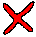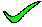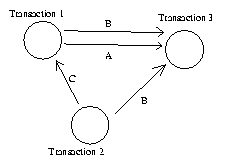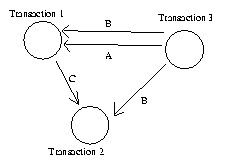To draw a presedence graph, you first draw three circles, one for each transaction. Next, you go through the schedule, drawing a line from Transaction A to Transaction B whenever;

• B reads an attribute which A has written to at a previous time
• B write an attribute which A has written to at a previous time
• B writes an attribute which A has read from at a previous time

The connection from Transaction 2 to 1 involving B, and from 3 to 1 involving B do not fall into any of these three categories.

closeTo draw a presedence graph, you first draw three circles, one for each transaction. Next, you go through the schedule, drawing a line from Transaction A to Transaction B whenever;

• B reads an attribute which A has written to at a previous time
• B write an attribute which A has written to at a previous time
• B writes an attribute which A has read from at a previous time

The graph shown has something missing.

closeTo draw a presedence graph, you first draw three circles, one for each transaction. Next, you go through the schedule, drawing a line from Transaction A to Transaction B whenever;

• B reads an attribute which A has written to at a previous time
• B write an attribute which A has written to at a previous time
• B writes an attribute which A has read from at a previous time

All the connections in this graph point the wrong way...

closeTo draw a presedence graph, you first draw three circles, one for each transaction. Next, you go through the schedule, drawing a line from Transaction A to Transaction B whenever;

• B reads an attribute which A has written to at a previous time
• B write an attribute which A has written to at a previous time
• B writes an attribute which A has read from at a previous time

The connection from Transaction 2 to 1 involving B, and from 3 to 1 involving B do not fall into any of these three categories. In addition, the remaining connections in this graph involve the correct attributes and transactions but point the wrong way...

closeTo draw a presedence graph, you first draw three circles, one for each transaction. Next, you go through the schedule, drawing a line from Transaction A to Transaction B whenever;

• B reads an attribute which A has written to at a previous time
• B write an attribute which A has written to at a previous time
• B writes an attribute which A has read from at a previous time

Well done.

close

 A B C D E TELL ME NEXT INDEX

Given the following transaction schedule:

timeTRANSACTION 1 TRANSACTION 2 TRANSACTION 3
1.2.3.4.